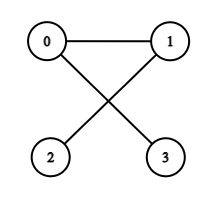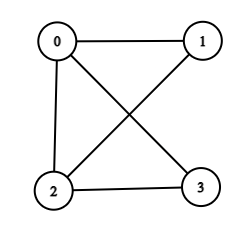New update is available. Click here to update.Close
Topic list
Is Graph Bipartite?
MEDIUM
Other topics
Problem solved
Skill meterStrings
-
-Matrices (2D Arrays)
-
--
-Sorting
-
-Binary Search
-
-Stacks & Queues
-
-Trees
-
-Graph
-
-Dynamic Programming
-
-Greedy
-
-Tries
-
-Arrays
-
-SQL
-
-Binary Search Trees
-
-Heap
-
-Bit Manipulation
-
-
Solve problems & track your progress
Checkout your overall progress in every topic here
BecomeSensei
in DSA topics
Open the topic and solve more problems associated with it to improve your skills
Check out the skill meter for every topic
See how many problems you are left with to solve for cracking any stage. Score more than zero to get your progress counted.

# Is Graph Bipartite?

Medium0/80Share0 upvotes

## Problem Statement

#### You are given an undirected graph consisting of ‘N’ nodes from 0 to ‘N’ - 1. You are given a list ‘EDGES’ of size ‘M’, consisting of all the edges of this undirected graph. Determine whether the given graph is Bipartite or not.

##### Note:
``````The graph has no self-edges, no parallel edges.

The graph may not be connected.

A graph is bipartite if the nodes of the graph can be partitioned into two independent sets A and B such that every edge in the graph connects a node in set A and a node in set B.
``````
##### For Example,
``````If ‘N’ = 4, ‘M’ = 5, edgeList = [ [0, 1],[0, 3],[1, 2] ].
````````````Here, you can see that the graph is bipartite as we can divide the nodes in two sets as follows:
setA = [0, 2].
setB = [1, 3].

In the graph, you can see that every edge in the graph connects a node in set A and a node in set B.
Hence, the output is “Yes”.
``````
Detailed explanation ( Input/output format, Notes, Constraints, Images )##### Sample Input 1 :
``````2
4 3
0 1
0 3
1 2
4 5
0 1
0 3
1 2
2 3
0 2
``````
##### Sample output 1 :
``````Yes
No
``````
##### Explanation For Sample Output 1:
``````For the first test case, the graph will be:
````````````Here, you can see that the graph is bipartite as we can divide the nodes into two sets as follows:
setA = [0, 2].
setB = [1, 3].

In the graph, you can see that every edge in the graph connects a node in set A and a node in set B.
Hence, the output is “Yes”.

For the second test case, the graph will be:
````````````Here, you cannot divide the nodes into two independent sets A and B such that every edge in the graph connects a node in set A and a node in set B. Hence, the output is “No”.
``````
##### Sample Input 2 :
``````2
4 4
0 1
0 2
0 3
2 3
3 3
0 2
1 0
1 2
``````
##### Sample output 2 :
``````No
No
``````AutoConsole